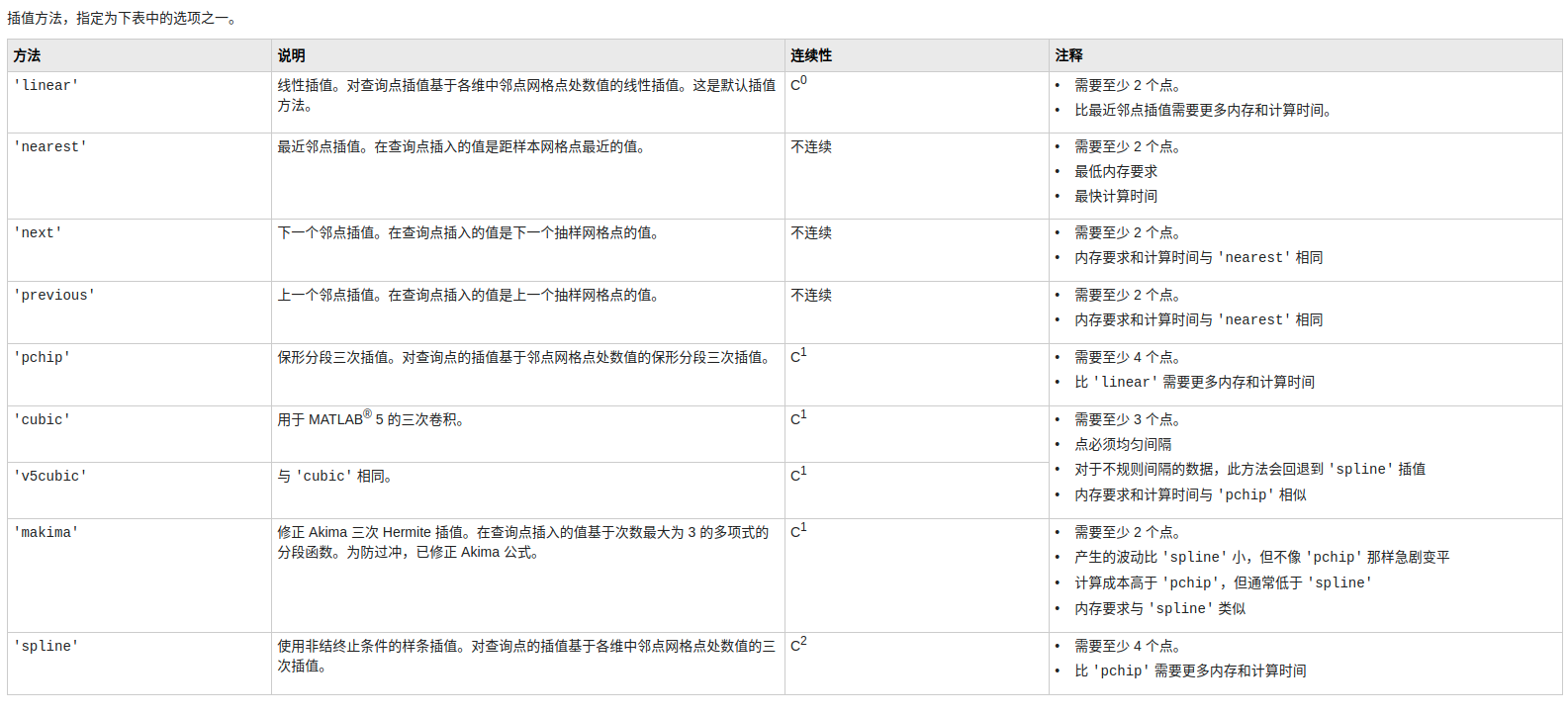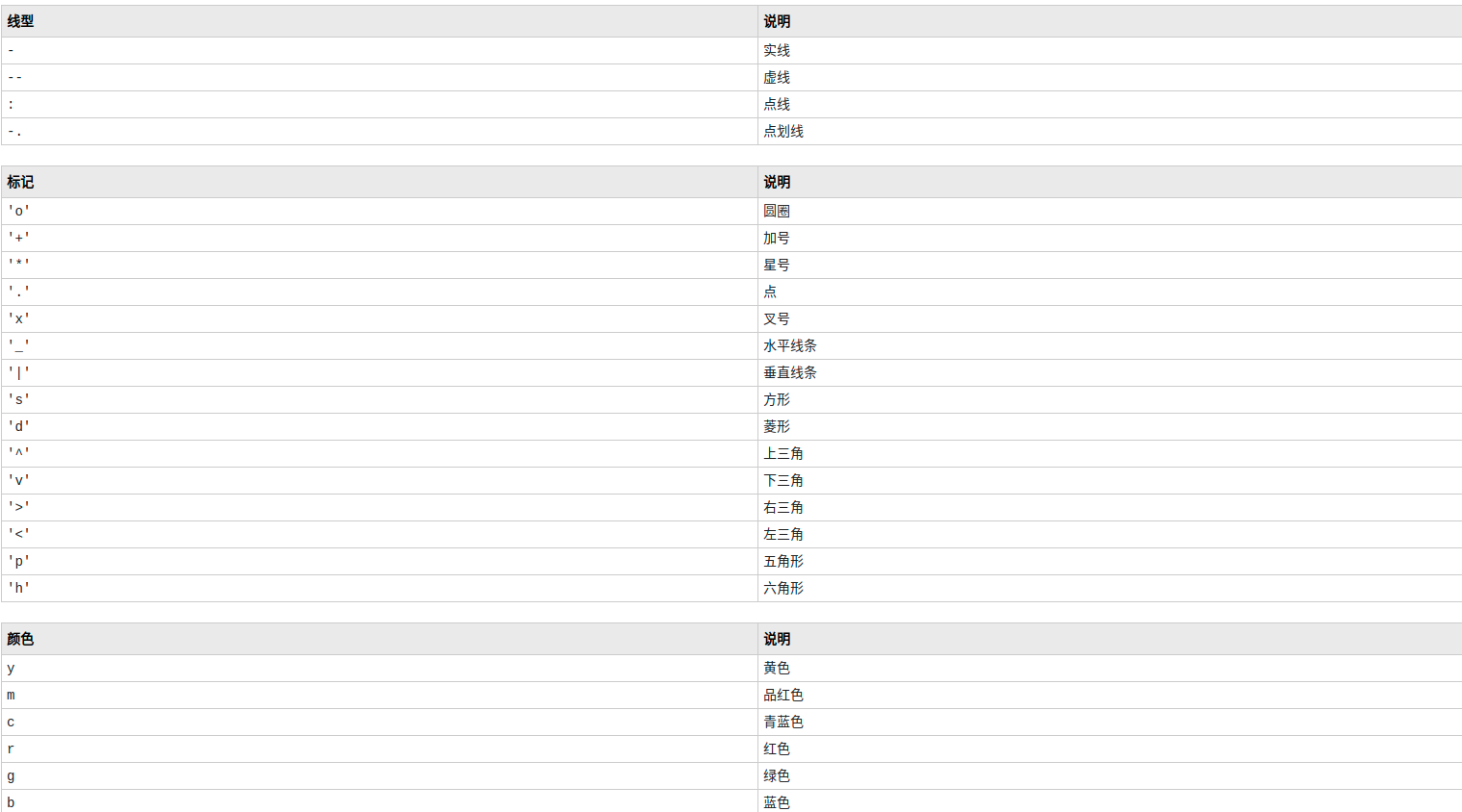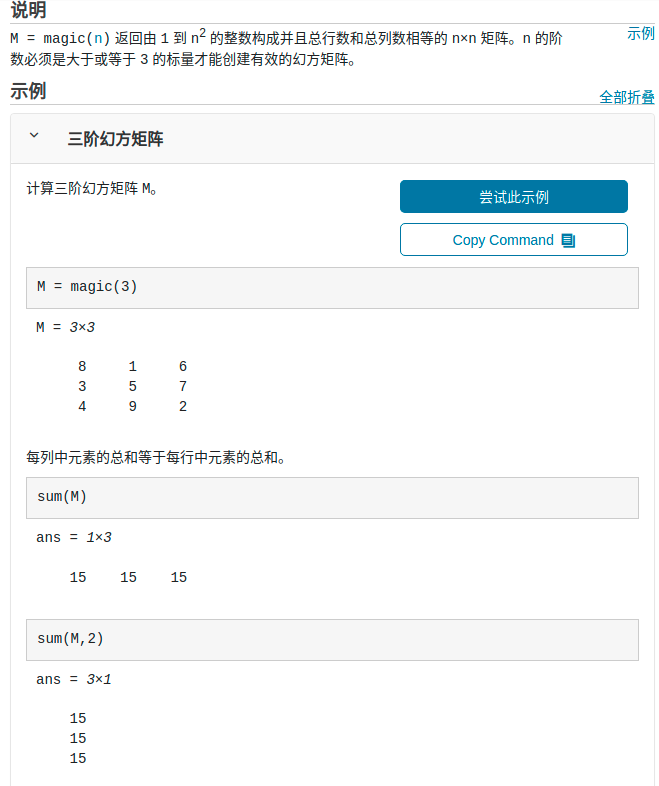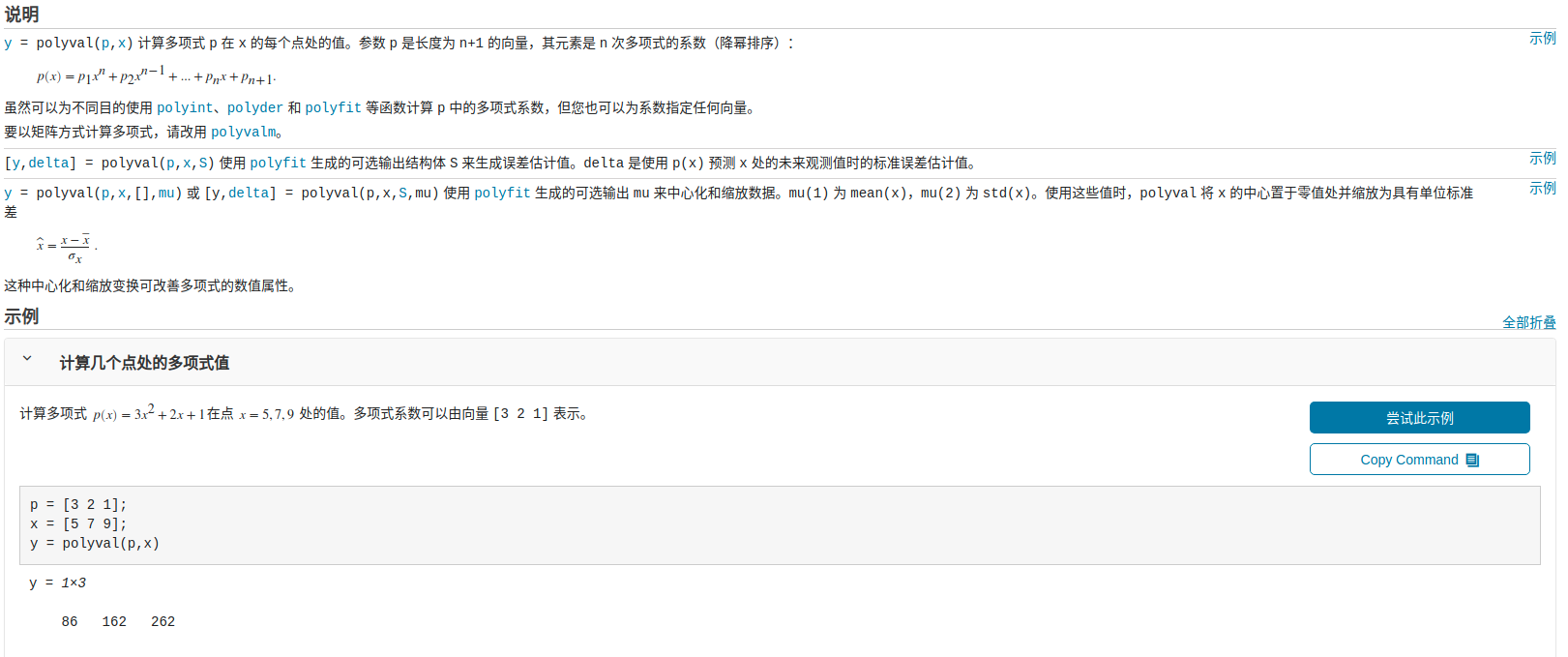# matlab期末速成笔记

​ 马上考matlab了，但是俺还是一点不会，在这里记一点笔记好了

• `int()`算积分的，第一个参数是式子，第二个是变量，后两个是积分区域

• `plot()`拿来画图像的

• `meshgrid(x,y)` 基于向量 `x``y` 中包含的坐标返回二维网格坐标。`X` 是一个矩阵，每一行是 `x` 的一个副本；`Y` 也是一个矩阵，每一列是 `y` 的一个副本。坐标 `X``Y` 表示的网格有 `length(y)` 个行和 `length(x)` 个列。

• `[X,Y] = meshgrid(x)``[X,Y] = meshgrid(x,x)` 相同，并返回网格大小为 `length(x)`×`length(x)` 的方形网格坐标。

• matlab中如果第一个if匹配成功就不会匹配下一个elseif

• `limit()`是拿来算积分的，(expr,x,a,b)

• `polyfit()`可以求方程的系数，第三个参数是多项式的次数

• `save xx`可以把变量保存为.mat文件

• `num2str()`用来数字转字符

• 同理，字符转数字是`str2num()`

• &适用于矩阵间的逻辑运算，&&不适用，只适用于单个元素，包括数值和字符

• 当判断两个逻辑值时，例如A&（f(x)>c）和A&&（f(x)>c）时，对于第一种情况，不论A是否为真，A和（f(x)>c）都要计算，但是对第二种情况，如果A是false，那么后面的（f(x)>c）就不用计算了，倘若f(x)是一个计算量大的函数，那么这将节省很多时间

• `interp1()`一维数据插值（表查找）

• vq = interp1(x,v,xq,method) 指定备选插值方法：’linear’、’nearest’、’next’、’previous’、’pchip’、’cubic’、’v5cubic’、’makima’ 或 ‘spline’。默认方法为 ‘linear’。

附文档的参数说明• 对于三角函数，直接用就行了，似乎没有用什么奇怪的函数名

• `'`用来转置矩阵，`.`让矩阵逐项运算

• `plot()` 画图的

诸如• 所谓魔方矩阵（magic matrix）就是值该矩阵有1~n2的正整数按照一定的规则排列而成，而且每一行、每一列、没条对角线上的元素都等于n(n2+1)/2。就生成规则而言魔方矩阵可以分为三类，①n为奇数，②n为不能被4整除的偶数，③n为能被4整除的偶数。

• 魔方函数`magic()`• max - 数组的最大元素
此 MATLAB 函数 返回数组的最大元素。 如果 A 是向量，则 max(A) 返回 A 的最大值。 如果
A 为矩阵，则 max(A) 是包含每一列的最大值的行向量。 如果 A 是多维数组，则 max(A) 沿
大小不等于 1 的第一个数组维度计算，并将这些元素视为向量。此维度的大小将变为 1，而所有其
他维度的大小保持不变。如果 A 是第一个维度长度为零的空数组，则 max(A) 返回与 A 大小相
同的空数组。

• sum - 数组元素总和
此 MATLAB 函数 返回 A 沿大小不等于 1 的第一个数组维度的元素之和。

• mean - 数组的均值
此 MATLAB 函数 返回 A 沿大小不等于 1 的第一个数组维度的元素的均值。

• std - 标准差
此 MATLAB 函数 返回 A 沿大小不等于 1 的第一个数组维度的元素的标准差。 如果 A 是观测
值的向量，则标准差为标量。 如果 A 是一个列为随机变量且行为观测值的矩阵，则 S 是一个包含
与每列对应的标准差的行向量。 如果 A 是多维数组，则 std(A) 会沿大小不等于 1 的第一个数
组维度计算，并将这些元素视为向量。此维度的大小将变为 1，而所有其他维度的大小保持不变。
默认情况下，标准差按 N-1 实现归一化，其中 N 是观测值数量。

标准差就是标准方差

• 直接`save PATH`可将全部变量保存

• `det()`拿来算行列式的

• INV - 矩阵求逆
此 MATLAB 函数 计算方阵 X 的 逆矩阵。

•• `y = linspace(x1,x2,n)` 生成 `n` 个点。这些点的间距为 `(x2-x1)/(n-1)`

`linspace` 类似于冒号运算符“`:`”，但可以直接控制点数并始终包括端点。“`linspace`”名称中的“`lin`”指示生成线性间距值而不是同级函数 `logspace`，后者会生成对数间距值。

• 将向量表示为以x为变量的多项式形式可以用`poly2str()`

• 在matlab中进行图形剪裁，实际上就是将对应的函数值设置为NaN

• matlab图形用户界面设计中用于建立控件对象的函数是`unicontrol`

• 在matlab命令窗口中>>标志为matlab的命令行提示符，|标志为输入提示符

• `addpath PATH -end`将PATH添加到matlab整个路径末尾

• 元胞数组是MATLAB中特有的一种数据类型，是数组的一种，其内部元素可以是属于不同的数据类型，概念理解上，可以认为它和c语言里面的结构体、c++里面的对象很类似。元胞数组是matlab中的特色数据类型，它不同于其它数据类型（如字符型，字符数组或者叫字符串，以及一般的算术数据和数组）。它特有的存取数据方法决定了它的特点，它有给人一种查询信息的感觉，可以逐渐追踪一直到所有的变量全部翻译成基本的数据信息。它的class函数输出就是cell（细胞之意）。

• matlab中用char(n)来定义，当然最基本的是包裹式定义，比如先定义了一个字符型的变量a，并赋值，然后定义一个长整型b，并赋值…最后用大括号来打包裹c={a,b}来形成元胞c，当然进一步可以将c再包裹进去如d={a,b,c,’abc’,123}都是合法的。

• 在元胞数组中，用()取数组的元素类型，用{}取数组的内容

• `subplot(m,n,p)` 将当前图窗划分为 `m`×`n` 网格，并在 `p` 指定的位置创建坐标区。MATLAB按行号对子图位置进行编号。第一个子图是第一行的第一列，第二个子图是第一行的第二列，依此类推。如果指定的位置已存在坐标区，则此命令会将该坐标区设为当前坐标区。

• 查看matlab工作空间的变量名，大小，尺寸和类型的命令为`whos`

• matlab中用系统预定义的特殊变量`eps`表示浮点数的精度。

• 如果被插值函数是一个单变量函数，则称为一维线性插值。

• 清除当前图形窗口内容的语句是`clf`

• 读取excel,`xlsread('PATH')`

• `size`的功能是确定矩阵的行数和列数

• 求多项式对应方程根，使用`roots()`,验证使用`polyval()`

• 预定义变量`nargin`用于记录调用函数时的输入参数个数

• 在创建图形对象时，都为该对象分配唯一的一个值，称之为图形对象的句柄

• 将未知长度的字符串向量a反向排列的语句是`a(end:-1:1)`

• 求两个多项式的乘积应该用`conv`,相除应该用`deconv`

• 图形用户界面设计中用于建立快捷菜单的函数是`unicontextmenu()`

• 条形图`bar`